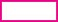# User ForumSubject :IMO    Class : Class 3

The difference between 456 tens andis 2456. What is the number in the box?

A 2912
B 2000
C 2104
D 7016

456 tens = 4560= 4560 - 2456= 2104

## Ans 1:

Class : Class 3
456 tens=4560-_=2456. You can find the answer by 4560-2456=2104.So the blank is =4560-2456=2104. This is the trick.

## Ans 2: (Master Answer)

Class : Class 1

(C): 456 tens = 4560

Now, it is given that

4560 –= 2456

∴= 4560 – 2456
∴= 2104

Class : Class 3